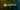# Functions in Python

105 Views

## Functions are the block of statements which are to be executed.

These are very useful as they are only implemented once and used many times for example if you want to perform a certain task for multiple times rather than writing, again and again, we use functions which makes our task easy.

Functions in python are created by using def keyword.

The variables which are defined inside the functions can be used only inside the functions as the scope is only inside the functions

For example:-

def functionExample():

print(“This is a Function”)

The above is an example of a function in python we can call the function by using the function name like in our case the function name is function example.

### How to call a function in python

Function example()

def function_example():

print(“This is an example of functions in python”)

function_example()

When you run the above code the output you see is “  This is an example of functions in python “

You can implement any number of functions as you need based on your requirements.

def function_example():

print(“This is an example of functions in python using loops”)

for i in range(5):

function_example()

Here we call the function inside the for loop so it will execute while the loop runs.

### Return keyword

The return keyword in python is used to return anything from the function we use return with value to return anything from the function.

We can also pass arguments to a function when calling a function.

So, it takes arguments and perform the task and return the result.

If the function definition is having parameters you have to call the function by using parameters.

You can define functions for multiple purposes like an easy way to understand your code and code readability.

Programme to find the addition of two numbers using python without using return : –

number1 = int(input(“Enter first number”))

number2 = int(input(“Enter second number”))

result = number1 + number2

print(result)

Programme to find the addition of two numbers using python using return : –

number1 = int(input(“Enter first number”))

number2 = int(input(“Enter second number”))

result = number1 + number2

return result

### Recursion in Python

Recursion is calling a function itself again and again.

In this type, the same function is called again for a certain number of times based on our requirements.

Recursion is a very important and powerful concept. Understanding recursion is very important as we can implement in very less number of lines.

Programme to find the factorial of a given number using recursion in python:-

number = int(input(“enter a number”))

def factorial(number):

if number==0 or number==1:

return 1

return number * factorial(number-1)

print(“The factorial of a given number is “, factorial(number))

Here we can see the function factorial called itself again and again to calculate the factorial. This is called recursion.

### Types of Functions

There are two types of functions in python like

Built-in Functions

User-defined Functions

Built-in functions are the functions that are built into python we only need to use them when we need that.

User-defined Functions are the functions which are created by us.

### Python lambda function

Lambda function is an anonymous function which means it cannot have a name.

Lambda functions are defined by using the lambda keyword.

Example of lambda function in python:-

result = lambda number : number * 2

print(result(10))

Here the number is the input while passing when calling that function. So, it will take input and compute the output.

### Global Variables in python

Global variables are the variables which can be used to access inside the functions and outside the functions or we can access them throughout all our programme.

Example :-

number = 12

def f1():

print(“Inside the function: “, number)

f1()

print(“outside the function: “, number)

Here number is accessed both inside and outside the function as it is defined outside.

### Local Variables

Local variables are the variables which are defined and used in a particular scope as if we declare any variable inside any function that variable can be accessed only inside the function which is local variable.

Example : –

def f1():

number = 12

print(“Inside the function: “, number)

f1()

Here the number cannot be accessed outside as it is defined inside the function which is local variable.

If we try to access the number outside the function it will give you an error as it defined inside the function the scope is only inside the function.